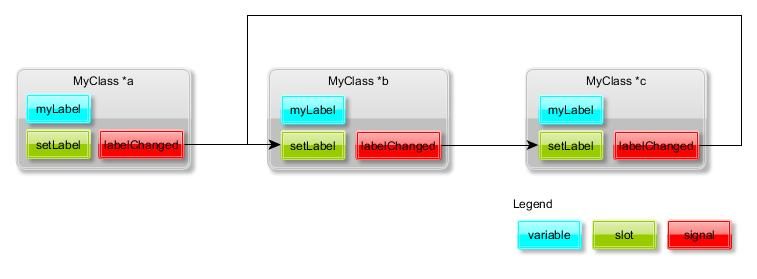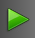# Signals and slots

I think now would be a good time to look briefly at the Qt signal and slot concepts. Basically signals and slots in Qt programming provide a method to connect objects together. I believe we should utilize this feature when passing information from QML to the Qt side and vice versa.

So here is a brief introduction to the topic.

Signals are declared inside a class declaration in the “signals” section. The signal is written as a method prototype (no implementation is needed for the method). Here is an example of a class that declares one signal called labelChanged. The signal can have one argument of type QString. Note also that the class must inherit the QObject class and should refer to the Q_OBJECT macro.

```#include <QObject>
class MyClass : public QObject
{
Q_OBJECT
signals:
void labelChanged(QString);
};
```

A slot is basically just a class method. It is declared in the “slots” section and then implemented like other methods. Let’s add one slot called setLabel to the MyClass declaration. The slot takes one argument of type QString that can be used to set the class variable myLabel.

```#include <QObject>
class MyClass : public QObject
{
Q_OBJECT
private:
QString myLabel;
signals:
void labelChanged(QString);
public slots:
void setLabel(QString label);
};
```

The slot must be implemented. The MyClass could be implemented as follows. Note that inside the setLabel slot we will “emit” our labelChanged signal (and pass the label string as an argument). This happens whenever the label value changes.

#include "myclass.h"
void MyClass::setLabel(QString label)
{
if( myLabel == label )
return;
myLabel = label;
emit labelChanged(myLabel);
}

Emitting signals does not have any effect unless we have connected the signal to one or more slots. The connection is done by calling a QObject method “connect”. E.g. in the main program we could connect two MyClass objects together like follows:

```    a = new MyClass();
b = new MyClass();
QObject::connect(a,SIGNAL(labelChanged(QString)),b,SLOT(setLabel(QString)));
```

If we are passing any arguments (like a QString in this case) we must of course make sure that the argument types will match between the signal and the slot. Now whenever the a-object emits the signal labelChanged the setLabel slot method in the b-object will be invoked and the QString argument will be passed to the b-object.

Ok, how about a working example?

Let’s construct a simple Qt console application where we connect three MyClass objects (or slightly enhanced versions of those shown above) together. The connections could be done like shown in the following figure (a connects to b, b connects to c and c connects back to b).Here is the class declaration (myclass.h). In addition to the signal and slot introduced earlier there is now also a constructor that names the class instance (sets the myName variable).

```#ifndef MYCLASS_H
#define MYCLASS_H

#include <QObject>
#include <QTextStream>

class MyClass : public QObject
{
Q_OBJECT
private:
QString myName;
QString myLabel;
public:
MyClass(QString text);
signals:
void labelChanged(QString);
public slots:
void setLabel(QString label);
};

#endif // MYCLASS_H
```

The class implementation (myclass.cpp) defines the class constructor and the slot.

```#include "myclass.h"

// Constructor
MyClass::MyClass(QString name)
{
myName = name;
}

void MyClass::setLabel(QString label)
{
if( myLabel == label )
return;

QTextStream(stdout) << myName << ": label changed: " << label << endl;
myLabel = label;
emit labelChanged(myLabel);
}
```

The main program does all the connections and then calls the setLabel slot (the slot can be called just like a normal class method). First the b-object setLabel is called which sets the label value to “First”. This will emit the b-object’s labelChanged signal which will propagate the change to the c-object. Note that even though the c-object will also emit a signal back to the b-object there will be no looping as the value does not change.

```#include "myclass.h"

int main()
{
MyClass *a, *b, *c;

a = new MyClass("a");
b = new MyClass("b");
c = new MyClass("c");

QObject::connect(a,SIGNAL(labelChanged(QString)),b,SLOT(setLabel(QString)));
QObject::connect(b,SIGNAL(labelChanged(QString)),c,SLOT(setLabel(QString)));
QObject::connect(c,SIGNAL(labelChanged(QString)),b,SLOT(setLabel(QString)));

QTextStream(stdout) << "Calling b->setLabel(\"First\")" << endl;
b->setLabel("First");

QTextStream(stdout) << "Calling a->setLabel(\"Second\")" << endl;
a->setLabel("Second");
}
```

Finally the a-object setLabel is called which will modify the myLabel variable value in all the three objects.

The output in the console window should look like this:

 Calling b->setLabel(“First”) b: label changed: First c: label changed: First Calling a->setLabel(“Second”) a: label changed: Second b: label changed: Second c: label changed: Second Press to close this window…

`https://github.com/n9dyfi/TestApp8.git`And to run the application: press the play button.